图像处理中的概率直方图_灰度图像的直方图 概率分布 - CSDN
• 图像处理大型科普——图像直方图偶尔跟在浙大读研的湖大一姐谈起轮廓识别，她正在做一个能把衣服分类成不同款式的应用，衣服平摊好，然后用手机一扫，马上就能告诉你这是T-SHIRT还是裙子，什么？你敢说这玩意鸡肋？T...
图像处理大型科普——图像直方图

偶尔跟在浙大读研的湖大一姐谈起轮廓识别，她正在做一个能把衣服分类成不同款式的应用，衣服平摊好，然后用手机一扫，马上就能告诉你这是T-SHIRT还是裙子，什么？你敢说这玩意鸡肋？T-SHIRT和裙子当然容易分别，但那些

莲蓬裙、连衣裙、连体裙、半截裙、背带裙、连体裤、热裤、短裤、超短裤、齐X裤、打底裤、靴裤、吊带裤你真的分得清楚么？

分不清的先去一旁罚歌一首继续说正事

学姐大概是这么做的，先灰度，然后获取高频分量，然后根据高频分量二值化，然后获取轮廓的点集，然后通过直方图跟数据库里的预设模型数据做相似度匹配，最后匹配度高的是结果。

慢着，直方图能做匹配？？

话刚码完，学姐刷的一下甩我一篇英文论文：“一起看看？”（论文的链接甩这：Shape-context.pdf）

在度娘、谷神以及有道哥的帮助下大致理解了文中的意思，也让我对直方图这个概念有了更深的认识

对于刚刚接触matlab图像处理的来说，一谈起直方图马上就想到了用imhist()绘制图像的直方图

又或者是用histeq()均衡直方图，让图片对比度更加高，甚至去除图片中的白噪（之前也写过一篇去除雾霾白噪的：MATLAB图像处理：一分钟去除图片中的雾霾  ）

尽管matlab中的直方图有很多现成的应用，但千万别把直方图的定义限制在这几个函数里了，难不成人家Word\Excel里的直方图就不是直方图了么？联想到各种报表、文案中的直方图，我觉得我们之所以使用直方图，主要是希望能有分类统计的功能，
对于图像处理中的直方图，对像素进行分类统计是我们最常使用的一种方式

于是得到灰度值的直方图：关于这张图，也是有很多地方可以用的到的

比如说我们可以利用图像的细长峰值判断图像的主要信息集中在哪个灰度上，

利用图像两峰之间的面积判断背景色在哪个灰度上，

也可以用峰谷判断噪点所在的灰度，

同样可以利用该图中的灰度期望给出一个理想的二值化分界值。

甚至在手绘（最近有点迷上painter手绘。。。）中决定绘画中的主色调，背景色，和高亮点所对应的色彩范围，大大减少配色的难度。

倘若把这个直方图量化开来，变成一个size = 256的[gray_value ,count_num]形式的矩阵，完全可以把它当做这张图片特有的指纹，一个256维的向量，无论这张图片旋转多少角度，这个向量都不会改变，是的，终于绕回来了，任意一张图片都可以对应这样的一个向量，并且维度都是256哦，至于向量，管他多少维的，都是一条直线，那么两条直线的相似度不就是直线的夹角咯，（此处不贴公式，想一探究竟的请自行百度“n维向量的夹角”）哈哈，这不就完美的解决了图片旋转前后的匹配的问题么~~

做事要严谨，我一开始的确以为这就是直方图的匹配方式的，不过仔细一想，256维的向量角度。。。。。这计算量，这精确度，还得经受环境光源的影响，这么苛刻的匹配环境用在识别衣服款式上，几乎是得不到回报的

回到上文中提到的论文中，还得怪我们对直方图的认识太肤浅狭隘，我们一直都在灰度值的直方图中打转转，用统计的思想去碰概率，然而直方图不仅是统计，还可以分类，不仅是灰度值的直方图，也可以是别的什么的直方图啊。

前方高能，集中注意

这里特别提到的，是用于分类 轮廓点集 的一种直方图：a和b是两张图的轮廓点集，c是一个坐标系，把一个圆按照离圆心的距离、和正北的角度两个指标分成60个块（角度分成12类区域，距离分成5类区域，12*5=60），然后遍历点集的每一个点，以当前遍历的点为C坐标的圆心，把其他点按60个区域划分，变成一个size = 60的素组，保存着每个块中的轮廓点数量，现在每个点都对应了一个关于其他点的直方图，就像d、e、f三张图一样，60个小方块，点的数量越多，颜色越深。

最后一步，比较a、b两两点间的相似度，获取匹配度最高的那个点与之对应，最终得运行结果就像图g中一样，无论图像旋转，缩放，还是光源的角度强弱，都不会对结果造成有效影响，最大程度上保证了匹配的精度。

这是一个很好的例子，来说明直方图在分类上的特别能力，我觉得在之后的应用中， 我们也可以通过制定分类指标的方式，把抽象的数据通过直方图分成大类，再进行简单的统计运算，最大化的发挥直方图在图相处理领域分类统计的作用。

希望大家通过这篇博文可以对身边的事物有更多奇葩的使用想法和理解方式，老是用别人的东西也没意思噻，反正这老外的思维我是服了

OVER~~
展开全文图像处理 matlab
• 直方图处理直方图直方图显示参考代码效果图与应用场景直方图均衡参考代码效果图与应用场景直方图规定参考代码效果图与应用场景局部直方图均衡参考代码效果图与应用场景直方图统计参考代码效果图与应用场景 直方图 ...
直方图处理直方图直方图显示参考代码效果图与应用场景直方图均衡参考代码效果图与应用场景直方图规定参考代码效果图与应用场景局部直方图均衡参考代码效果图与应用场景直方图统计参考代码效果图与应用场景
直方图
直方图是图像像素灰度级的统计数目的直观表示。本文将从像素级操作完成直方图显示、直方图均衡、直方图规定（匹配）、直方图局部均衡以及直方图统计测试实验。
直方图显示
对于直方图的计算，我们需要统计各个灰度级上像素的数目，然后根据此关系勾画出直方图。直方图能够直观的显示图像像素在各个灰度级的分布，是其余实验的基础。
参考代码
#include<iostream>
#include<string>
#include<opencv2/core/core.hpp>
#include<opencv2/highgui/highgui.hpp>
#include<opencv2/opencv.hpp>
using namespace cv;
using namespace std;
void test1();
double* computeHist(Mat src);
void showHist(double* rank, string name);
double findNumber(double* num, int start, int end, int n);
void test2();
Mat myBalanceHist(Mat &src);
double* standHist(double* rank);
double* balanceHist(double* rank);
Mat mapping(Mat &src, double* rank);
void test3();
void standardImageSingleArray(Mat &src, double* num, int rank);
void gama_image(Mat &img, double gama, double rate);
Mat myMatchHist(Mat &src, double* dstHist);
void test4();
Mat myLocalBalance(Mat &src, int size);
void test5();
Mat statisticHist(Mat &src, double rate, double muk0, double sigmak1, double sigmak2, bool dark, int size);
Mat countMV(double* num, int nums);
int main()
{
test1();
test2();
test3();
test4();
test5();
return 0;
}

// 测试一
void test1()
{
// 读取灰度图像
// 显示直方图
double* rank = computeHist(img);
showHist(rank, "原图直方图");
imshow("原图", img);
waitKey(0);
destroyAllWindows();
}

// 计算直方图
double* computeHist(Mat src)
{
double* rank = new double;
// 初始化
for (int n = 0; n < 256; n++)
rank[n] = 0;
// 计算灰度分布
for (int i = 0; i < src.rows; i++)
for (int j = 0; j < src.cols; j++)
{
rank[src.at<uchar>(i, j)]++;
}
return rank;
}

// 显示直方图
void showHist(double* rank, string name)
{
Mat hist = Mat::zeros(255, 255, CV_8UC1);
// 深拷贝
double* rankCopy = new double;
for (int n = 0; n < 256; n++)
rankCopy[n] = rank[n];
double min = findNumber(rankCopy, 0, 256, 0);
double max = findNumber(rankCopy, 0, 256, 255);
for (int i = 0; i < 256; i++)
{
line(hist, Point(i, 255), Point(i, saturate_cast<int>(255 - (rank[i] - min) * 255 / (max - min))), Scalar(255));
}
imshow(name, hist);
}

// 寻找序列值
double findNumber(double* num, int start, int end, int n)
{
int p = start;
double stand = num[start];
for (int i = start + 1; i < end; i++)
{
if (num[i] < stand)
{
num[p] = num[i];
p++;
num[i] = num[p];
}
}
if (p == n)
return stand;
else if (p > n)
return findNumber(num, start, p, n);
else
return findNumber(num, p + 1, end, n);
}

效果图与应用场景直方图能够让观察者对图的灰度分布有一个直观的认识，方便后续图像增强工作的处理。

直方图均衡
直方图均衡是根据变换函数 $s = (L-1)\int_{0}^{r}w(r)\text{d}w$ 来进行映射的。原理大概可解释为在任意间隔内的灰度密度等于灰度间隔除以总灰度级，那么这个灰度分布就是均衡的。
$s+k = (L-1)\int_{0}^{r}w(r)\text{d}w+(L-1)\int_{r}^{r+t}w(r)\text{d}w$
$\frac{k}{L-1} =\int_{r}^{r+t}w(r)\text{d}w$
等式右边为灰度级从k到s+k所映射的像素占比为$\frac{k}{L-1}$，得证。
参考代码
// 测试二
void test2()
{
// 读取灰度图像
double* rank = computeHist(img);
Mat dst = myBalanceHist(img);
// 显示均衡后的直方图
double* rankBalance = computeHist(dst);
showHist(rank, "原图像直方图");
showHist(rankBalance, "均衡后的直方图");
imshow("原图", img);
imshow("直方图均衡", dst);
waitKey(0);
destroyAllWindows();
}

// 直方图均衡化
Mat myBalanceHist(Mat &src)
{
double* rank;
rank = computeHist(src);
// 归一化直方图
rank = standHist(rank);
// 直方图均衡
balanceHist(rank);
// 图像映射
Mat dst = mapping(src, rank);
return dst;
}

// 归一化直方图
double* standHist(double* rank)
{
// 深拷贝
double* rankCopy = new double;
for (int n = 0; n < 256; n++)
rankCopy[n] = rank[n];
double sum = 0;
for (int n = 0; n < 256; n++)
sum += rankCopy[n];
for (int n = 0; n < 256; n++)
rankCopy[n] /= sum;
return rankCopy;
}

// 直方图均衡
double* balanceHist(double* rank)
{
// 深拷贝
double* rankCopy = new double;
for (int n = 0; n < 256; n++)
rankCopy[n] = rank[n];
for (int i = 0; i < 256; i++)
{
for (int j = 0; j < i; j++)
rank[i] += rankCopy[j];
rank[i] *= 255;
}
return rank;
}

// 映射
Mat mapping(Mat &src, double* rank)
{
Mat dst = src.clone();
for (int i = 0; i < src.rows; i++)
for (int j = 0; j < src.cols; j++)
{
dst.at<uchar>(i, j) = saturate_cast<int>(rank[src.at<uchar>(i, j)]);
}
return dst;
}

效果图与应用场景对比原图与均衡后的图像，可发现变换图像的层次效果更加明显，直方图的分布基本占据整个灰> > 度级且各灰度级密度基本相当，而存在空缺的灰度级是因为图像灰度级是离散的，变换中存在多> > 个灰度级映射到同一个灰度级。

直方图均衡是自适应的，不需要对不同图像进行特定的调整，因此使用比较方便，应用场景较宽泛，多用于图像的预处理，增强图像的对比度。

直方图规定
直方图规定，或者称直方图匹配，算是直方图均衡的变种。直方图均衡化后图像的直方图是均匀分布的，而直方图规定化后图像的直方图分布是可控制的，因此需要提供一个各个灰度级的概率密度数据来进行匹配。
该算法的实现原理：

先对原图像进行直方均衡化，可得到一种映射关系$f(x)$；再对输入的灰度密度数据进行直方图均衡化，又可以得到一种映射关系$g(x)$。之后求$g(x)$的反函数$g^{-1}(x)$，可得到映射关系$f(g^{-1}(x))$，即原图像到目标概率密度的映射。但实际上灰度级是离散的，多个灰度级映射到一个灰度级上，$g(x)$可能是递增的但不是严格单调递增的，反函数可能不存在，这种情况需要进行一些特殊的处理，$g^{-1}(x)$如果输出对应多个则映射到最小值，如果输出不存在，则对应到差值最小的存在的灰度级上。

参考代码
// 测试三
void test3()
{
// 读取灰度图像
Mat dst = img.clone();
gama_image(dst, 2.5, 1);
// 绘制直方图
double* rankImg = computeHist(img);
double* rankDst = computeHist(dst);
// 直方图规定
Mat matchDst = myMatchHist(img, rankDst);
// 绘制规定的直方图
double* rankMatch = computeHist(matchDst);

showHist(rankImg, "原图直方图");
showHist(rankDst, "伽马直方图");
showHist(rankMatch, "规定直方图");
imshow("原图", img);
imshow("伽马变换", dst);
imshow("直方图规定", matchDst);
waitKey(0);
destroyAllWindows();
}

// 单通道图像标定——数组存储
void standardImageSingleArray(Mat &src, double* num, int rank = 255)
{
// 查找数组内的最大值与最小值
double min = 1000000000, max = -1000000000;
for (int i = 0; i < src.rows; i++)
for (int j = 0; j < src.cols; j++)
{
if (num[i*src.cols + j] < min)
min = num[i*src.cols + j];
if (num[i*src.cols + j] > max)
max = num[i*src.cols + j];
// cout << num[i*src.cols + j] <<endl;
}

// 对图像像素值进行标定
for (int i = 0; i < src.rows; i++)
for (int j = 0; j < src.cols; j++)
{
src.at<uchar>(i, j) = saturate_cast<int>(rank * ((num[i*src.cols + j] - min) / (max - min)));
}
}

void gama_image(Mat &img, double gama, double rate = 1)
{
double* num = new double[img.rows*img.cols];
int n = 0;
// 初始化图像迭代器
MatIterator_<uchar> srcIterStart = img.begin<uchar>();
MatIterator_<uchar> srcIterEnd = img.end<uchar>();

while (srcIterStart != srcIterEnd)
{
if (*srcIterStart == 0)
num[n] = 1.0;
else
num[n] = rate * pow(*srcIterStart, gama);
n++;
srcIterStart++;

}
standardImageSingleArray(img, num);

}

// 直方图规定化
Mat myMatchHist(Mat &src, double* dstHist)
{
// 均衡映射数组
double* srcRank = computeHist(src);
srcRank = standHist(srcRank);
srcRank = balanceHist(srcRank);
// 均衡规定映射数组
double* dstRank = standHist(dstHist);
dstRank = balanceHist(dstRank);

double* rank = new double;

for (int i = 0; i < 256; i++)
for (int j = 0; j < 256; j++)
{
if (srcRank[i] >= dstRank[j])
if (abs(srcRank[i] - dstRank[j]) <= abs(srcRank[i] - dstRank[j + 1]) || j == 255)
rank[i] = j;
}
Mat dst = src.clone();
dst = mapping(dst, rank);
return dst;
}

效果图与应用场景本测试， 使用伽马变换后的图像灰度分布当做规定模板，对比伽马变换图与规定直方图可知，两者区别不大，本次实验成功。直方图规定化可应用在指定灰度分布的作业上。

局部直方图均衡
全局直方图均衡是在整个图像中做一次均衡化， 而局部直方图均衡是在给定的模板大小的像素邻域内进行均衡化，有多少像素就得进行多少次均衡化，计算量较大。
参考代码
// 测试四
void test4()
{
// 读取灰度图像
Mat globalDst = myBalanceHist(img);
Mat localDst = myLocalBalance(img, 3);

imshow("原图", img);
imshow("直方图均衡", globalDst);
imshow("局部直方图均衡", localDst);
waitKey(0);
destroyAllWindows();
}

// 局部直方图均衡 ——待优化
Mat myLocalBalance(Mat &src, int size = 3)
{
Mat dst = src.clone();
for (int i = size / 2; i < (src.rows - size / 2); i++)
for (int j = size / 2; j < (src.cols - size / 2); j++)
{
// 初始化
double* num = new double;
for (int p = 0; p < 256; p++)
num[p] = 0;

for (int m = -size / 2; m <= size / 2; m++)
for (int n = -size / 2; n <= size / 2; n++)
{
num[dst.at<uchar>(i + m, j + n)]++;
}

num = standHist(num);
num = balanceHist(num);
dst.at<uchar>(i, j) = saturate_cast<int>(num[dst.at<uchar>(i, j)]);

delete[]num;
}
return dst;
}

效果图与应用场景局部直方图均衡可应用于显示局部对比度不强，而全局对比度又足够的图像，来获取局部的细节。

直方图统计
直方图统计也是根据模板大小内的像素邻域的均值、方差与全局的均值、方差的比较，来决定像素的操作。操作暗亮区域的图像只需要设置局部均值和全局均值的比值，而局部方差一般设置成小于全局的方差。
参考代码
// 测试五
void test5()
{
// 读取灰度图像
Mat darkDst = statisticHist(img, 10, 0.5, 0.02, 1, true, 3);
Mat lightDst = statisticHist(img, 0.1, 0.5, 0.02, 1, false, 3);
Mat globalDst = myBalanceHist(img);

imshow("原图", img);
imshow("直方图统计暗区域", darkDst);
imshow("直方图统计亮区域", lightDst);
imshow("直方图均衡", globalDst);
waitKey(0);
destroyAllWindows();
}

// 直方图统计
Mat statisticHist(Mat &src, double rate, double muk0, double sigmak1, double sigmak2, bool dark = true, int size = 3)
{
Mat dst = src.clone();
double mean, variance, lmean, lvariance;
double* num = new double[src.rows*src.cols];
double* lnum = new double[size*size];
int point;
for (int i = 0; i < src.rows; i++)
for (int j = 0; j < src.cols; j++)
num[i*src.rows + j] = src.at<uchar>(i, j);
Mat xy = countMV(num, src.rows*src.cols);
mean = xy.at<double>(0, 0);
variance = xy.at<double>(1, 0);
delete []num;

for (int i = size / 2; i < (src.rows - size / 2); i++)
for (int j = size / 2; j < (src.cols - size / 2); j++)
{
point = 0;
for (int m = -size / 2; m <= size / 2; m++)
for (int n = -size / 2; n <= size / 2; n++)
{
lnum[point] = dst.at<uchar>(i + m, j + n);
point++;
}
xy = countMV(lnum, size*size);
lmean = xy.at<double>(0, 0);
lvariance = xy.at<double>(1, 0);
if (dark)
{
if (lmean < muk0*mean && lvariance > sigmak1*variance && lvariance < sigmak2*variance)
dst.at<uchar>(i, j) = saturate_cast<int>(rate * dst.at<uchar>(i, j));

}
else
{
if (lmean > muk0*mean && lvariance > sigmak1*variance && lvariance < sigmak2*variance)
dst.at<uchar>(i, j) = saturate_cast<int>(rate * dst.at<uchar>(i, j));
}
}
return dst;
}

// 计算均值和方差
Mat countMV(double* num, int nums)
{
double sum = 0, mu, sigma;
for (int i = 0; i < nums; i++)
{
sum += num[i];
}
mu = sum / nums;

sum = 0;

for (int i = 0; i < nums; i++)
{
sum += pow(num[i] - mu, 2);
}
sigma = sum / nums;
Mat xy = (Mat_<double>(2, 1) << mu, sigma);
return xy;
}

效果图与应用场景直方图统计可指定灰度且对比差的区域进行相应的提升。


展开全文数字图像处理 cpp opencv 图像增强
• 数字图像处理直方图处理直方图处理概念直方图均衡化（Histogram Equalization）直方图规定化局部直方图处理 直方图处理概念 灰度级范围为[0,L-1]的数字图像的直方图是离散函数h（rk）=nk，其中rk是第k级灰度值，nk...
数字图像处理之直方图处理直方图处理概念直方图均衡化（Histogram Equalization）直方图规定化（Histogram Specification）局部直方图处理
直方图处理概念
灰度级范围为[0,L-1]的数字图像的直方图是离散函数h（rk）=nk，其中rk是第k级灰度值，nk是图像中灰度为rk的像素个数。在实践中，经常用乘积MN表示的图像像素的总数除它的每个分量来归一化直方图，通常M和N是图像的行和列的维数。因此，归一化后的直方图由p（rk）=nk/MN给出，其中k=0,1,…,L-1。简单来说，p（rk）是灰度级rk在图像中出现的概率的一个估计。归一化直方图的所有分量之和应等于1。
在直方图中，暗图像集中分布在灰度级的低端；亮图像集中分布在灰度级的高端。低对比度图像具有较窄的直方图，且集中于灰度级的中部；高对比度图像的直方图分量则覆盖了很宽的灰度级范围。
直方图均衡化（Histogram Equalization）
均衡化的基本原理：是把原始图的直方图变换为均匀分布的形式，这样就增加了象素灰度值的动态范围从而可达到增强图像整体对比度的效果。
均衡化步骤：

统计图像中每个灰度级出现的次数，计算图像中每个灰度级出现的概率；
根据变换公式得到直方图均衡化的变换函数；
根据变换函数映射到每个像素点；
输出映射后的图像；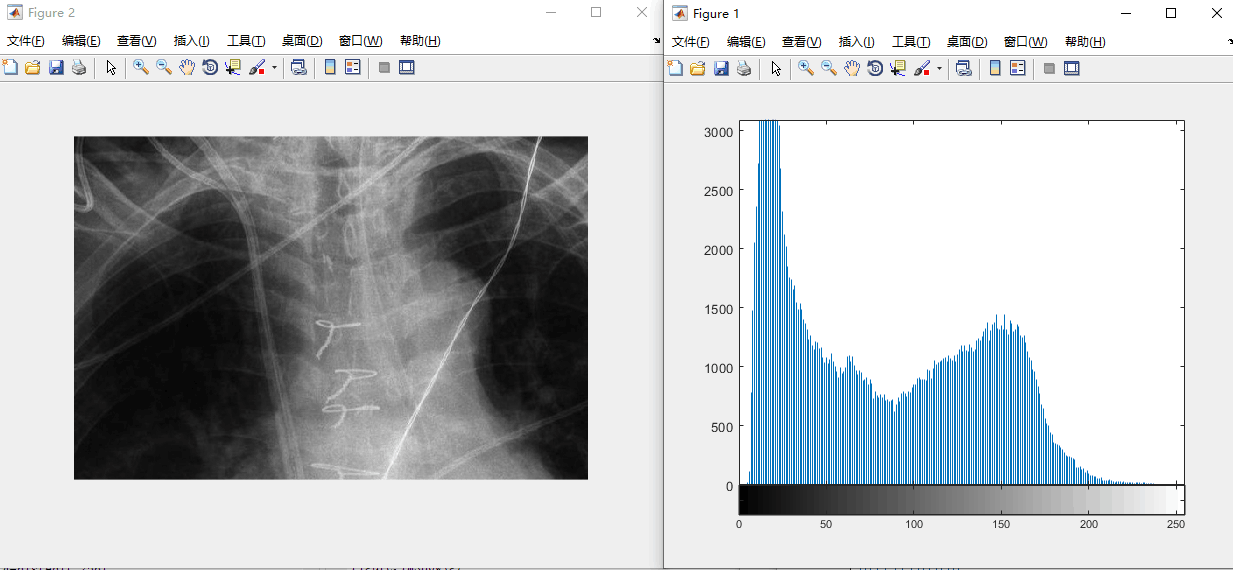均衡化之前的图像

clear all
>> f=rgb2gray(f);
>> imshow(f);
>> imhist(f);
figure,imshow(f);
g=histeq(f,256);
figure,imhist(g);
figure,imshow(g);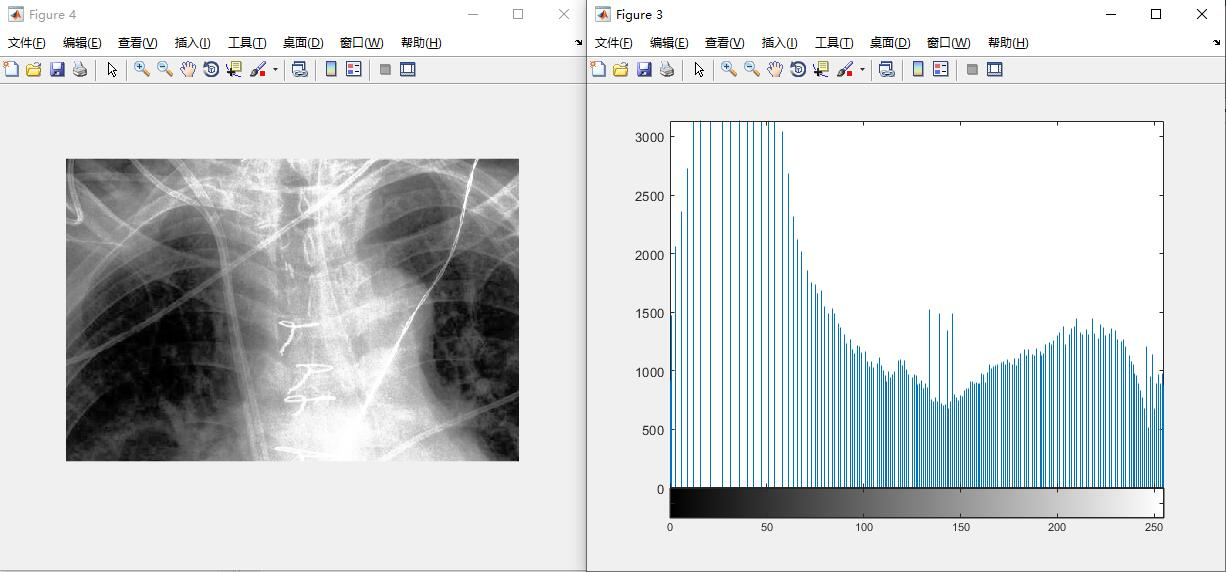均衡化之后的图像
直方图规定化（Histogram Specification）
规定化的基本原理：有目的的增强某个灰度区间的图像，即能够人为地修正直方图的形状，使之与期望的图像相匹配。
规定化步骤：
计算给定图像的直方图pr®，并用它寻找直方图均衡变化，把sk四舍五入为范围[0，L-1]内的整数；
计算变化函数G的所有值，把变换函数G的值四舍五入为范围[0，L-1]内的整数，并将G的值放入一个表中；
对每一个值sk，使用步骤2存储的G值寻找相应的zq值，以使G（zq）最接近sk，并存储这些从s到z的映射。当满足给定sk的zq值多余一个时（即映射不唯一时），则选择最小的值；
首先对输入图像进行均衡，然后用步骤3找到的映射把该图像中的每个均衡后的像素值sk映射为直方图规定化后的图像中的相应zq的值，形成直方图规定化后的图像。
clear all
r=127;
x=-r:r+1;
sigma=20;
y1=exp(-((x-80).^2)/(2*sigma^2));
y2=exp(-((x+80).^2)/(2*sigma^2));
y=y1+y2;                        %双峰高斯函数，任意函数都可以

y=y/sum(y);         %归一化，使函数符合概率分布的sum(y)==1这样一个规律
plot(y);            %待匹配的直方图

G=[];               %函数的累积直方图
for i=1:256
G=[G sum(y(1:i))];
end

img=rgb2gray(img);
[m n]=size(img);
hist=imhist(img);       %待处理图像的直方图
p=hist/(m*n);
figure;plot(p)          %原图直方图

s=[];                   %待处理图像的累积直方图
for i=1:256
s=[s sum(p(1:i))];
end

for i=1:256
tmp{i}=G-s(i);
tmp{i}=abs(tmp{i});         %因为要找距离最近的点，所以取绝对值
[a index(i)]=min(tmp{i});   %找到两个累积直方图距离最近的点
end

imgn=zeros(m,n);
for i=1:m
for j=1:n
imgn(i,j)=index(img(i,j)+1)-1;    %由原图的灰度通过索引映射到新的灰度
end
end

imgn=uint8(imgn);
figure;imshow(imgn)
figure;plot(imhist(imgn))       %新图的直方图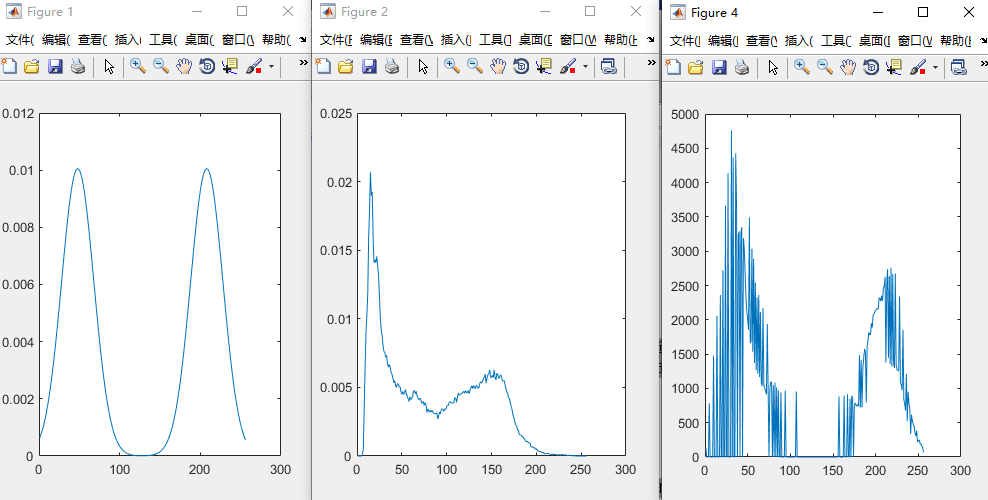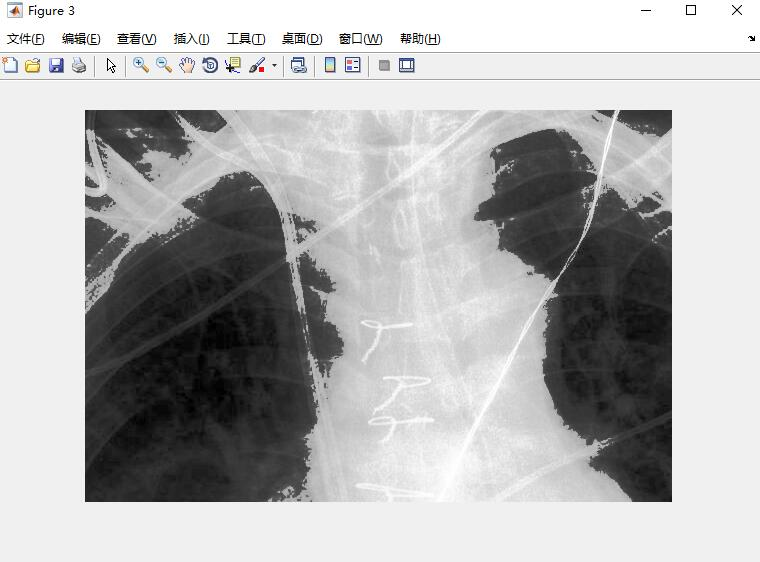局部直方图处理
在某种意义上，像素被基于整幅图像的灰度分布的变换函数修改。虽然这种全局方法适用于整个图像的增强，但存在这样的情况，增强图像中小区域的细节也是需要的。这些区域中，一些像素的影响在全局变换的计算中可能被忽略了，因为全局变换没有必要保证期望的局部增强。解决方法是以图像中每个像素的邻域中的灰度分布为基础设计变换函数。
前面描述的直方图处理技术很容易适应局部增强。该过程是定义一个邻域，并把该区域的中心从一个像素移至另一个像素。在每一个位置，计算邻域中的点的直方图，并且得到的不是直方图均衡化，就是规定化变换函数。这个函数最终用于映射邻域中心像素的灰度。然后，邻域中心的被移至一个相邻像素位置，并重复该过程。当邻域进行逐像素平移时，由于只有邻域中的一行或一列改变，所以可在每一步移动中，以新数据更新前一个位置得到的直方图。这种方法与区域每移动一个像素位置就计算邻域中所有像素的直方图相比有明显的优点。有时用于减少计算量的另一种方法是使用非重叠区域，但这种方法通常会产生我们不希望的“棋盘”效应。


展开全文• 灰度级范围为[0,L-1]的数字图像直方图是离散函数： 其中是第k级灰度值(=k)，是图像中灰度值为的像素个数。 通常用MN表示的图像像素的总数除它的每个分量来归一化直方图，即： M和N分别是图像的行和列维数，...
灰度级范围为[0,L-1]的数字图像的直方图是离散函数：

其中是第k级灰度值(=k)，是图像中灰度值为的像素个数。

通常用MN表示的图像像素的总数除它的每个分量来归一化直方图，即：

M和N分别是图像的行和列维数，k = 0,1,...,L-1。归一化直方图的所有分量和为1。

若一幅图像的像素倾向于占据整个可能的灰度级并且均匀分布，则该图像会有高对比度的外观和灰度细节丰富的特点。如下图：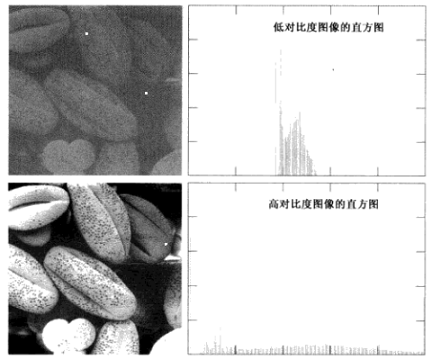直方图均衡

用变量r表示待处理的图像的灰度，灰度变换函数通常需要满足两个条件：

(a) T(r)在空间上为单调递增函数
(b) 当时，
当考虑反函数时，条件(a)改为：

(a') T(r)在空间上是严格单调递增函数
条件(a)保证输出灰度值不少于相应的输入值，防止灰度反变换时产生人为的缺陷；条件(b)保证输出灰度的范围与输入灰度的范围相同；条件(a')保证从s到r的反映射是单值的，即一对一的，防止出现二义性。如下图：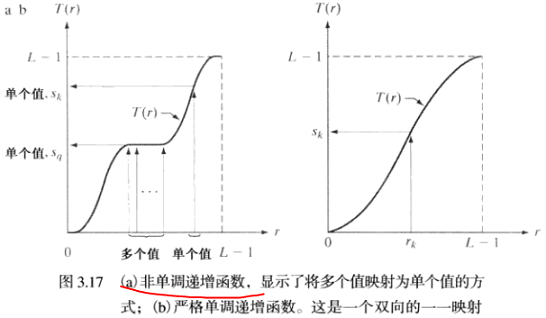为了产生均衡的直方图，使用如下的灰度变换函数：

....................................(1)

容易看出，该变换满足条件a和b。

下面解释为什么经过(1)式的灰度变换能产生均衡直方图。

令和分别表示随机变量r和s的概率密度函数(PDF)。若和T(r)已知，则变换后的变量s的PDF为：

......................................................(2)

使用(1)式的变换函数，得：

将dr/ds代入(2)式，得：

可知，始终是一个均匀概率密度函数，与的形式无关。所以，经过(1)式灰度变换的图像能产生均衡直方图。

(1)式的积分形式针对的是连续灰度值，而对于离散值，用求和的形式处理。一幅图像中灰度级出现的概率为：

(1)式中变换的离散形式为：

注：在实际使用中，需要将灰度值取近似为最接近的整数。

直方图匹配（规定化）

直方图匹配或直方图规定化，是指处理后的图像具有规定的直方图形状。

具体说来就是，要找到一个灰度变换函数，使图像在该灰度变换函数的作用下，产生一个我们指定形状的直方图，所谓指定形状的直方图形状，其实就是一个指定的概率密度函数。

假设r和z分别表示输入图像和输出图像的灰度级，是输入图像的概率密度函数，是我们希望输出图像所具有的指定概率密度函数。

令：

可以看出，这就是直方图均衡中的变换函数。知道，就能得到T(r)。

定义随机变量z：

同样，知道，就能得到G(z)，而就是我们指定的概率密度函数。

可得：

上式就是我们要求的直方图匹配的灰度变换函数。

对于离散形式，类似的我们令：

其中，规定的直方图的第i个值，是规定的直方图对应的图像的第q级灰度值（=q），利用反变换找到期望的：

实践中，并不需要反变换。因为我们处理的灰度级是整数（如8比特图像的灰度级是从0到255），我们常用下面的直方图规定化过程：

计算给定图像的及其直方图均衡变换，把四舍五入为范围[0,L-1]内的整数；
对q=0,1,2,...,L-1计算，其中规定的直方图的值，把G四舍五入为范围[0,L-1]内的整数，将G存在一个表中；
对每一个，k=0,1,2,...,L-1，从步骤2中存储的G值表中寻找使最接近。例如表中第64个最接近，则根据的定义，此时q=63，也就是=63。当满足给定的值多于一个时，按惯例选择最小的值。

展开全文图像处理 图像直方图处理 直方图均衡 直方图规定化
• 图像增强中我们也可以使用直接从直方图中获得的统计参数。 1、平均值、方差的定义 令r表示在区间[0 , L-1]上代表离散灰度的离散随机变量，并且令表示对应于的归一化直方图分量，我们可以把看做是灰度级出现的...
• 灰度直方图灰度直方图是灰度级的函数，描述的是图像中具有该灰度级的像元的个数。确定图像像元的灰度值范围，以适当的灰度间隔为单位将其划分为若干等级，以横轴表示灰度级，以纵轴表示每一灰度级具有的像元数或该像...数字图像处理 直方图处理
• 直方图就是对数据进行统计，将统计值组织到一系列事先定义好的bin(直方图中的柱子)中。bin中的数值是从数据中计算出的特征的统计量，这些数据可以是诸如梯度、方向、色彩或任何其他特征。无论如何，直方图获得的是...图像处理 扩展
• ## 图像直方图与直方图均衡化

万次阅读 多人点赞 2016-01-29 12:47:05
图像直方图直方图均衡化图像直方图以及灰度与彩色图像直方图均衡化图像直方图：概述：图像直方图用来表征该图像像素值的分布情况。用一定数目的小区间(bin)来指定表征像素值的范围,每个小区间会得到落入该小区...计算机视觉 python 图像直方图 直方图均衡化 线性插值
• 数字图像处理--直方图均衡化直方图均衡化涉及变量核心原理均衡过程图示 作为帮助自己理解的笔记，不甚严谨，谨慎参考。 直方图均衡化 涉及变量 r：灰度变换处理前的像素值。 T( r )：把像素值r映射到像素值s的...数字图像处理
• 直方图 ...直方图均衡化就是将一已知概率密度分布的图像，经过某种变换变成一幅均匀概率密度分布的新图像，其结果为扩展了象元取值的动态范围，从而达到增强图像对比度的效果 直方图均衡化原理 ht...直方图均衡
• 图像直方图归一化 图像直方图概念： 图像直方图是反映一个图像像素分布的统计表，其实横坐标代表了图像像素的种类，可以是灰度的，也可以是彩色的。纵坐标代表了每一种颜色值在图像中的像素总数或者占所有像素个数的...MATLAB 数字图像处理 图像直方图
• ## 《数字图像处理》--直方图处理

万次阅读 多人点赞 2016-10-08 20:44:02
直方图灰度直方图是灰度级的函数，描述的是图像中具有该灰度级的像元的个数。确定图像像元的灰度值范围，以适当的灰度间隔为单位将其划分为若干等级，以横轴表示灰度级，以纵轴表示每一灰度级具有的像元数或该像元数...直方图处理 数字图像处理
• 如果我们要谈论局部直方图处理，那么不得不提起与之相对应的全局直方图处理，之前写过一篇文章关于全局直方图处理的，对全局直方图不熟悉的话可以先去看一下，这样再看局部直方图处理会更好。 当我想让一幅图想的...opencv 计算机视觉 算法 c++
• 本文主要介绍了灰度直方图相关的处理，包括以下几个方面的内容： 利用OpenCV计算图像的灰度直方图，并绘制直方图曲线 直方图均衡化的原理及实现 直方图规定化（匹配）的原理及实现 图像的灰度直方图 一幅图像由...
• 1.直方图规定化 所谓直方图规定化，就是通过一个灰度映像函数，将原灰度直方图改造成所希望的直方图。...由于都是作直方图均衡化处理，所以处理后的原图像的灰度概率密度函数Ps（S）及理想图像的灰度概率...
• 图像处理8：直方图均衡化 (1)概率密度函数： ①概率密度函数简介: 在数学,连续型随机变量的概率密度函数是一个描述这个随机变量在某个确定的取值点附近的可能性的函数。而随机变量的取值落在某个区域之内的...图像处理
• 数字图像处理实验（1）——直方图均衡化 实验题目： 编程实现灰度和彩色图像的直方图均衡化处理。要求给出原始图像的直方图、均衡化图像及其直方图直方图均衡化时所用的灰度级变换曲线图。注意彩色图像需要编程...计算机视觉 图像识别
• 图像直方图表示图像中每一灰度级与该灰度级出现的像素或频数间的统计关系，其数学公式表达如下： (10-1)P(rk)=nkN,(k=0,1,2,...,L−1) P(r_k) = \frac{n_k}{N},(k=0,1,2,...,L-1) \tag{10-1} P(rk​)=Nnk​​,(k...图像处理 直方图均衡化 图像增强 人工智能
• 图像处理中绘制图像直方图往往是观察和处理图像的利器之一。 直方图的观察方面的基本知识： 横坐标代表着灰度级、纵坐标是该灰度值在图像出现的概率或者次数。 直方图的型态为斜态和峰态，斜态指的是直方图的不...图像处理 python
• 图像直方图均衡化 首先，我们要理解什么是图像直方图均衡化： 把原始图像的灰度直方图从比较集中的某个灰度区间变成在全部灰度范围内的均匀分布。直方图均衡化就是对图像进行非线性拉伸，重新分配图像像素值，使一定...MATLAB 数字图像处理 图像直方图均衡化
...